# Sample Data: Childhood Leukaemia Lymphoma

Locations of childhood leukaemia and lymphoma annotated with case/control marks

## Details

Locations of childhood leukaemia and lymphoma in a polygonal observation region bounded by the region Rectangle[{4690., 4150.}, {5411., 4758.}] * 100 meters, annotated with case/control marks.

## Examples

### Basic Examples (1)

 In:=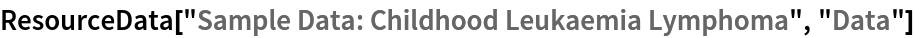Out=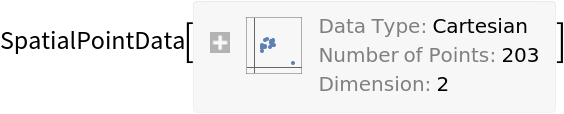Summary of the spatial point data:

 In:=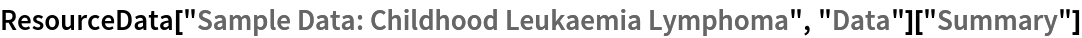Out=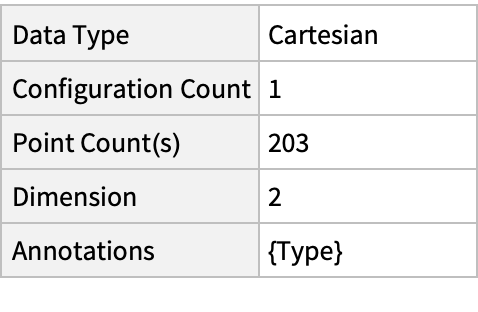### Visualizations (2)

Plot the spatial point data:

 In:=Out=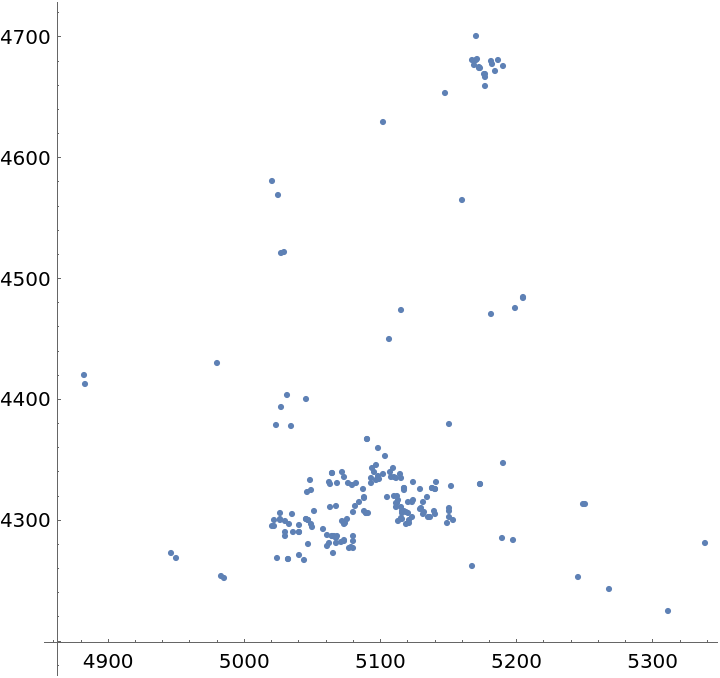Plot the points with type annotations:

 In:=Out=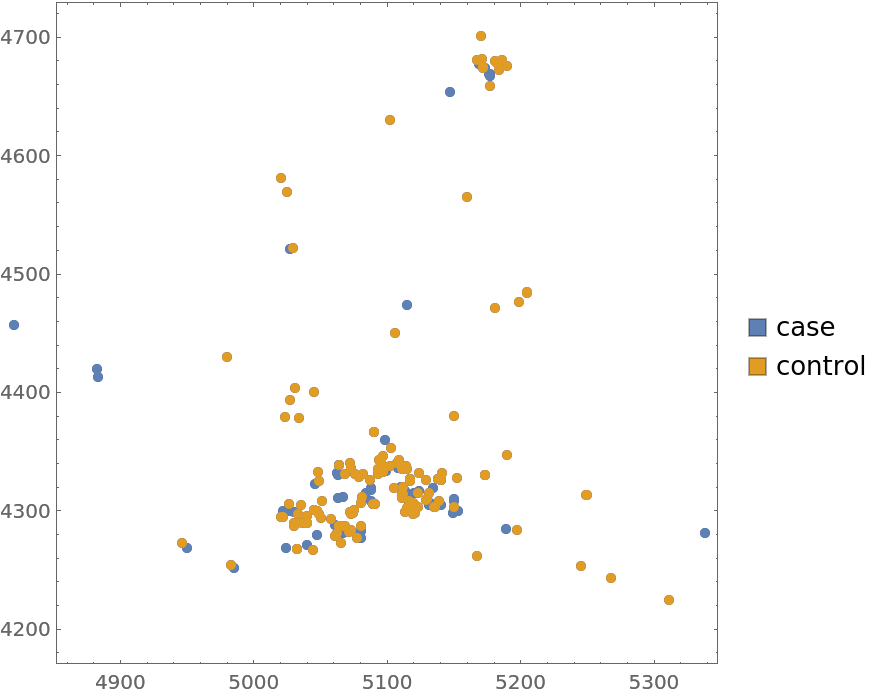### Analysis (3)

Compute probability of finding a point within given radius of an existing point - NearestNeighborG is the CDF of the nearest neighbor distribution:

 In:=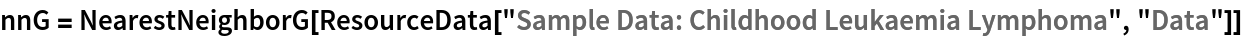Out=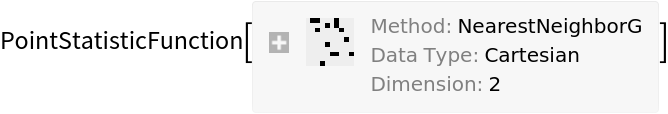In:=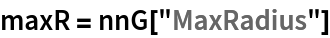Out=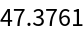In:=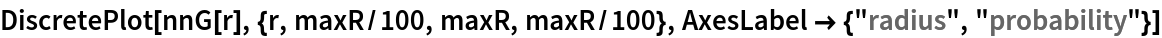Out=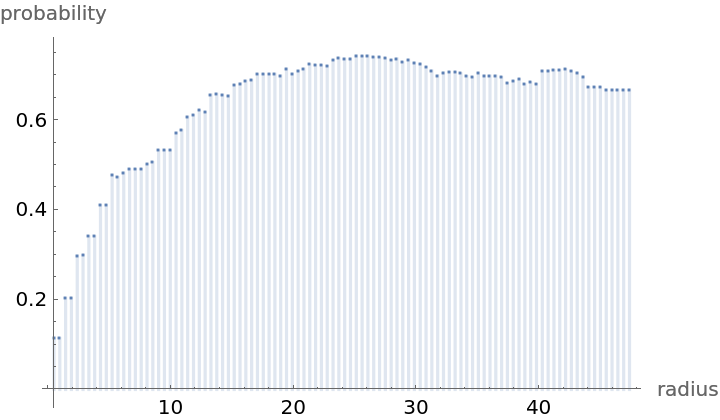Mean distance between a typical point and its nearest neighbor (for positive support distribution can be approximated via a Riemann sum of 1-CDF):

 In:=In:=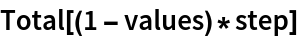Out=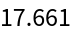Test for complete spacial randomness:

 In:=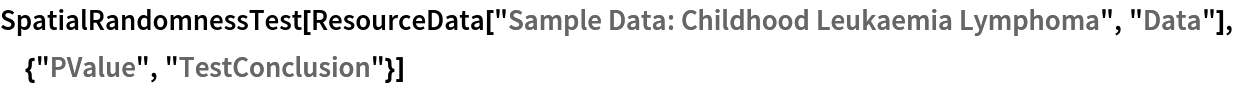Out=Gosia Konwerska, "Sample Data: Childhood Leukaemia Lymphoma" from the Wolfram Data Repository (2021)